# Beispiel einer Messung Behringer NX1000 Amplifier

### SMPTE

This method of audio analysis has also been standard in the Burosch NF Lab.. This test involves subjecting the device under test (DUT) to a low frequency tone (usually 60 Hz) and a mid-high tone (usually 7 KHz) and looking at the output. The ratio is also important, and the lower frequency tone is usually the stronger stimulus signal, and is usually 12 dB higher than the higher frequency tone.

### ITU-R

The ITU method might be even older than the SMPTE measurement, though it was originally known as the CCIF method. While the SMPTE was created by motion picture engineers, the CCIF/ITU test was created by communication engineers. In this test, two tones are generated 1 KHz apart and at the same amplitude. Usually, this is 19 KHz and 20 KHz, and then the resulting 1 KHz tone level is measured and referenced to the combined amplitude of the 19 and 20 KHz tones. But there are other variants of the test where the second and third order products are considered, or the 2nd through 5th order products are considered. The 1 KHz measurement is easy to make on the QA400.

In setting up to make an SMPTE IMD measurement, we’ll use both generators on the QuantAsylum Audioanalyzer QA400. Set the first generator to 60 Hz and 0 dBV, and the second generator at 7 Khz and –12 dBV (recall the SMPTE spec is that the higher frequency tone was 1/4 the level of the lower frequency tone, and 1/4th is the same as -12 dB).

In the plot below, we can see the frequency and amplitude of the tones is correct. In the green box, you can see the tone at 7 KHz has some mixing products appearing. If we turn off the 60 Hz tone, the spectrum around 7 KHz gets clear. These are indeed mixing products.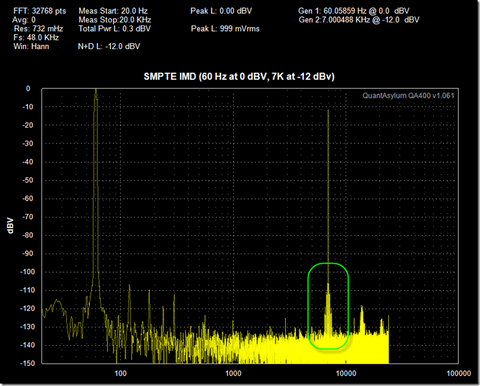If we zoom in on the 7 KHz tone, we can see the products more clearly. And indeed, measuring with markers reveals these are 60 Hz modulation products. In computing the IMD products, we’re interested in comparing the modulation products relative to the carrier, or 7 KHz tone. In the graph below, we can see a single modulation product is –106.7 dB below the carrier. There’s another on the other side at the same level. These two would sum and increase the overall level by 6 dB of this particular pair.

How far out you go generally depends on how quickly the harmonics fall off. The SMPTE spec is interested in ALL of the energy outside the 7 KHz tone, and thus it’d be correct to sum them all together. But you can get fairly accurate by simply considering the first pair or two. In this case, the harmonics are 100.7 dB below the carrier, and thus the SMPTE IMD figure would be –100.7 dB or 10^(-100.7/20) * 100 = 0.000922%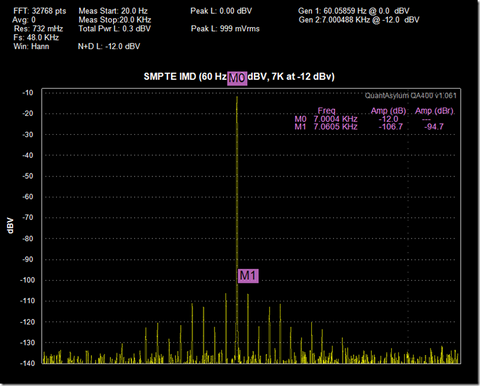To make the basic ITU measurement, we set the two generators to 19 KHz at –6 dBV and 20 KHz at –6 dBV, respectively. These two tones will sum to 0 dBV, which will also be the reference stimulus for this measurement. Remember, for the ITU test, the levels will almost always be equal.Above, we can see the resulting 1 KHz mixing product. Remember, this is called a mixing product because it is the difference between the two stimulus frequencies (19 KHz and 20 KHz). There are other mixing products too. And which ones you consider are a function of the flavor you have chosen for the IMD test. In more advanced instances of this test, you might also considering the following:

1 * F1 +/- 1 * F2 = 19 +/- 20 = 39KHz and 1 KHz (2nd order IMD)

2 * F1 +/- 1 * F2 = 38 +/- 20 = 58 KHz, 18 KHz (3rd order)

1 * F1 +/- 2* F2  & 1 = 19 +/- 40 = 59 KHz and 21 KHz (3rd order)

2 * F1 +/- 2* F2 = 38 +/- 40 = 78 KHz and 2 KHz (4th order)

3 * F1 +- 2 * F2 = 57 +/- 40 = 97 KHz and 17 KHz (5th order)

2 * F1 +/- 3 * F2 = 38 +/- 60 = 98 KHz and 22 KHz (5th order)

For the simple case, we’ll consider just the 1 KHz resultant 2nd order IMD product. Here, with a 0 dB reference and a 1 KHz product at –113.4 dB, the resulting ITU IMD is –113.4 dB, or 10^(-113.4/20) * 100 = 0.000213%. To properly put this measurement in context when sharing the result, it’s very important to specify the stimulus frequencies and that only the resultant 1 KHz tone was considered.

In the plot above, we can clearly see the other frequencies around the ~20 KHz signals. Zooming in shows more detail. We can see the 18 KHz product (3rd order) and 17 KHz product (5th order). A reasonable measurement to make would be a 2nd and 3rd order measurement. In this case, we’d want to sum resulting 2nd and 3rd order harmonics and compare those to the stimulus.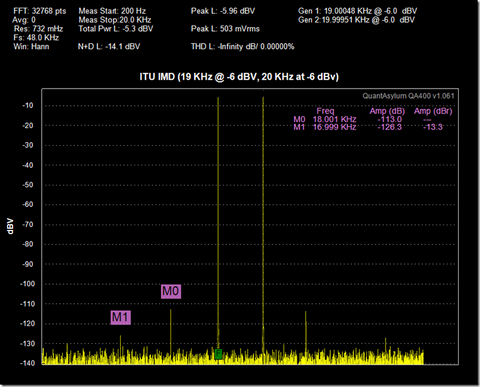### THD vs. POWER

Next, we're going to make a graph of the amplifier's THD versus output Power. We need to turn off the A-Weighting that was enabled in the noise test above. To sweep THD versus power, we'll use a plugin selected as shown below: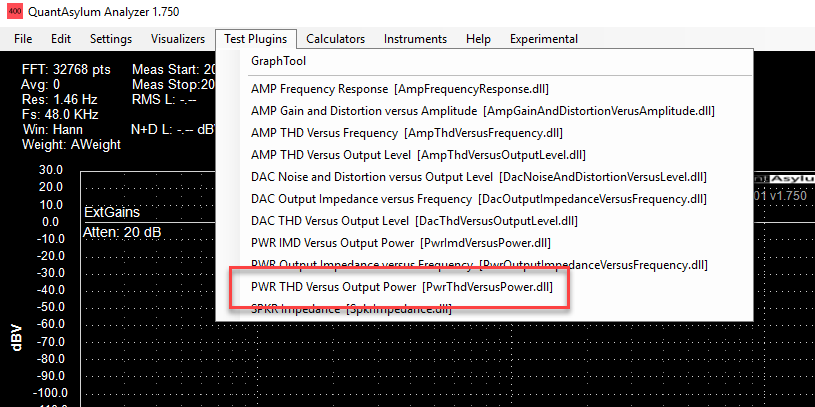The configuration screen for that appears as follows.

The settings for the various entries will change depending on the amp. What this test will do as configured above is as follows:The amp will sweep a 1 kHz tone from -30 dBV to 0 dBV. The power output will be calculated based on the specified load impedance and specified external gain. These settings are very important to have correct!

The attenuator will be managed automatically. What this means is the test will automatically engage the attenuator when needed, and where that occurs depends on whether you are measuring THD or THD+N. The test will also automatically stop IF the measured power exeeds 10W AND the THD exceeds -40 dB. This will protect the amp and load, and should be used anytime you are dealing with an unfamiliar amp. Running the test as configured above yields the following graph. The test was first run at volume midpoint, and then repeated at max volume.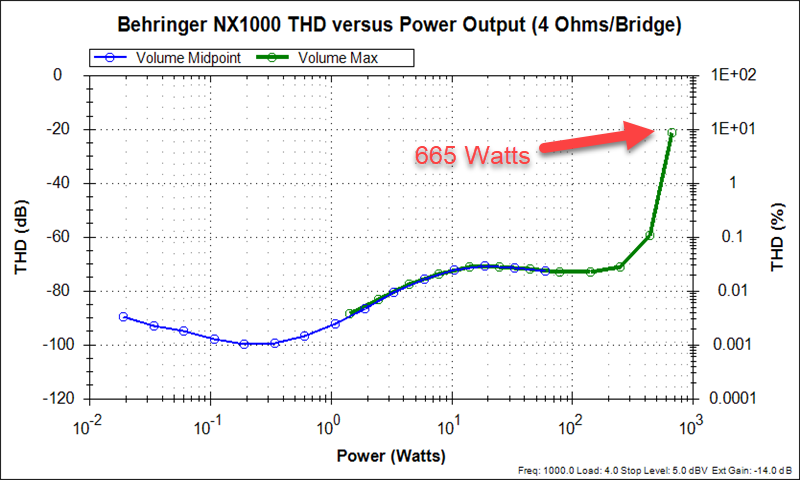From the plot above, we can see there is reasonably continuity between the two volume settings. In other words, you aren't penalized from a THD perspective by leaving the volume at max all the time (although you will pay a penalty in noise floor).

Finally, let's take a look at the spectrum at max volume and 192 kSps so that we can look out to nearly 100 kHz. The output level was adjusted until just before the THD started to degrade quickly, which is around the 350W point.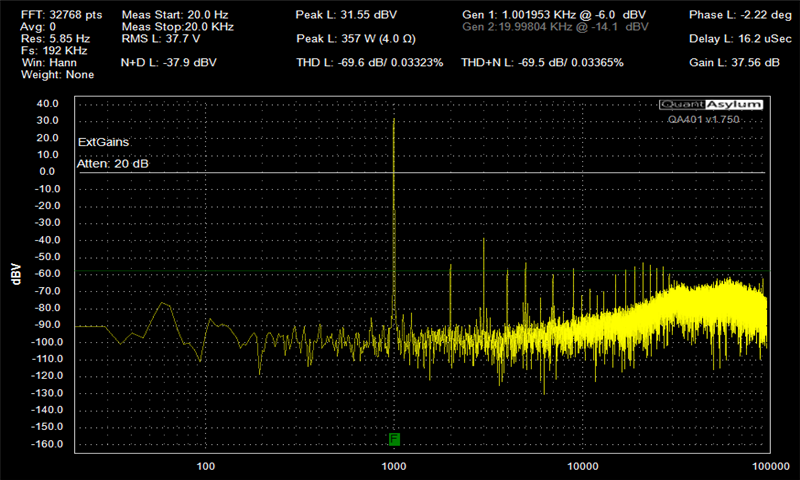Note the level of gain provided by the amp: about 37.6 dB. Overall the spectrum above looks well behaved out to 100 kHz, and is absent any spurious tones that might harm high frequency drivers. Given that the amp is rated at 1000W in bridge mode, and that our measurements showed the 10% distortion point being hit at 665W, it seems a stretch to claim this is a 1000W amp. For commercial audio, an amp should achieve the rated power at 1% THD 24x7, with peaks beyond that hitting 10%.

This post walked through the concerns and considerations of measuring a high-power/high-gain class D amplifier, and how you can ensure your system is setup correctly to give correct measurements.

The Behringer NX1000 is a discrete realization of a class D amp. It has a clever feature that was tested but not documented in this post where it adapts its frequency response to the load. And it works. That is a shortcoming shown previously in the TPA3255 amp post. But considering TI's TPA3255 hits 600W at 10% and this NX1000 hits about 670W at 10%, it's tough to justify the NX1000's discrete effort at this power level. Probably the reason a Behringer engineer might give is that their current discrete architecture provides them a path up to thousands of watts (side note: there's a good write-up here on amp power levels required for live music) . That allows them to tier their amplifiers by the number of MOSFETs in the power stage. Something that couldn't be done with current class D chipamp.

When working with high power amplifiers, it's easy to overstress the DUT or the load. The most common cause for this is not understanding the gains of each component in the signal chain, or incorrectly entering a gain as an attenuation (or vice versa). Move slowly when you are bringing up a new DUT, and sanity check your first measurements with a DVM. It takes 60 seconds, and is a sure-fire way to diagnose incorrect setup parameters in your measurements.

The THD spec on the QA401 is -108.5 dB, measured in loopback mode, single ended, L- shorted to ground, attenuator off, 32K FFT, Hann windowing, 48 KSPS and -10 dBV.

Because the measurement is made in loopback mode, it's hard to distinguish where the DAC performance ends and the ADC performance begins. In a previous blog post, we took a look distortion limits of the QA401 when using a notch filter.  In this post, we'll take a look at some sweeps to see where the sweet spot is for the QA401 in loopback mode. In this first plot, you can see a THD sweep from -22 dBV to -8 dBV. Best case THD appears around -14 dBV and 2 KHz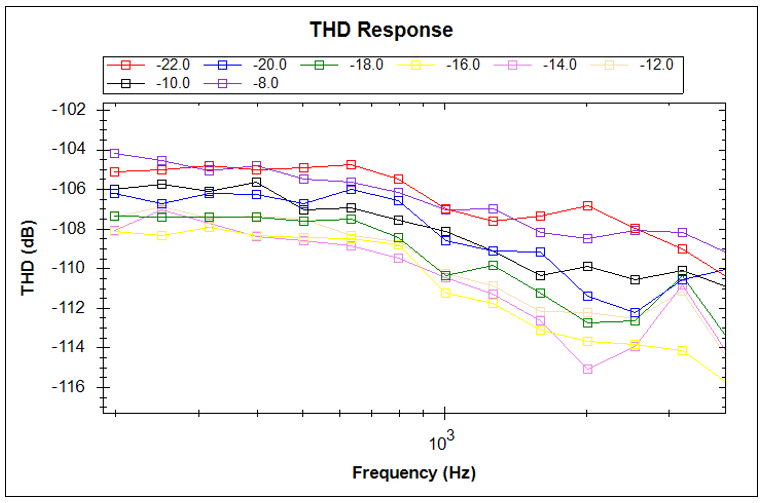Focusing on that operating point, we see the graph below. Using markers, we can see the first harmonic at 4 KHz is -120 dB below the fundamental, which is exceptional. The measured THD at this point is -115.7 dB--considerably better than the spec'd value for the QA401 of -108.5 dB. Of course, some QA401 may be slightly better and some may be slightly worse than this -115.7 dB figure.At 1 KHz, the optimal point for 1 KHz operation we can read off the swept plot is at -16 dBV. Below is a plot of that measurement:While not as impressive as the 2 KHz point, the above shows that the second and third harmonics are both about 120 dB below the fundamental.